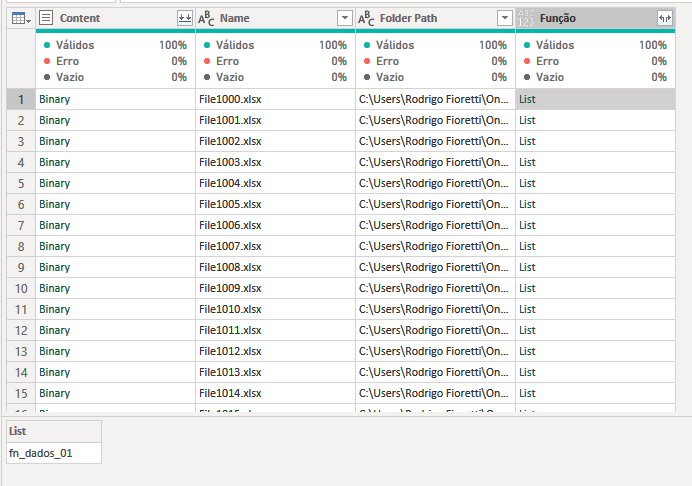cancel
Showing results for
Did you mean:Frequent Visitor

## Invoke Custom Function

Hello,

I have different versions for xlsx files, and I’ve created custom function for each version in order to group them.

Before I had more than one version for those xlsx, I had just one function to transform each xlsx and it was working very well. I could invoke it as figure 02 shows.

Now I have one function that indicates with function name power query should invoke to transform the file xlsx, but I can figure out how to invoke it. Figure 03.

Is there any way to call the custom function using a columns value as a parameter?Figure 01 - Column that indicates which function to invoke.Figure 02 – Previous query. Works fine for same xlsx versions.Figure 03 – Current query. Can’t figure out how to invoke the function from column [Função].

2 ACCEPTED SOLUTIONSSolution Sage

Hi @r_fioretti,

A couple of things I want to call out:

Table.TransformColumns doesn't allow field access beyond the the column you're transforming, instead you can use Table.ReplaceValue.

Inside the [Funcao] I see a list with what appears to be a textual string, not a function. You'll need to access the list and if it is a string pass Expression.Evaluate as well.

Here's an example that illustrates both scenario's:

``````let
fxEven = (n) => n+1,
fxOdd  = (n) => n+1,
Source = Table.FromColumns(
{{1..9}},
type table [ n =number]
),
ReplaceWithFunction = Table.ReplaceValue(AddListWithFunction,each [n],each Function.Invoke( [Fx]{0}, {[n]} ),Replacer.ReplaceValue,{"n"}),
ReplaceWithText = Table.ReplaceValue(AddListWithText,each [n],each Function.Invoke( Expression.Evaluate( [Txt]{0}, [fxEven2 = (n) => n+1, fxOdd2  = (n) => n+1] ), {[n]} ),Replacer.ReplaceValue,{"n"})
in
ReplaceWithText``````

Ps. If this helps solve your query please mark this post as Solution, thanks!Frequent Visitor

As you said Table.TransformColumns doesn't allow field access, so I added a new column instead of transforming the [Content] column.2 REPLIES 2Solution Sage

Hi @r_fioretti,

A couple of things I want to call out:

Table.TransformColumns doesn't allow field access beyond the the column you're transforming, instead you can use Table.ReplaceValue.

Inside the [Funcao] I see a list with what appears to be a textual string, not a function. You'll need to access the list and if it is a string pass Expression.Evaluate as well.

Here's an example that illustrates both scenario's:

``````let
fxEven = (n) => n+1,
fxOdd  = (n) => n+1,
Source = Table.FromColumns(
{{1..9}},
type table [ n =number]
),
ReplaceWithFunction = Table.ReplaceValue(AddListWithFunction,each [n],each Function.Invoke( [Fx]{0}, {[n]} ),Replacer.ReplaceValue,{"n"}),
ReplaceWithText = Table.ReplaceValue(AddListWithText,each [n],each Function.Invoke( Expression.Evaluate( [Txt]{0}, [fxEven2 = (n) => n+1, fxOdd2  = (n) => n+1] ), {[n]} ),Replacer.ReplaceValue,{"n"})
in
ReplaceWithText``````

Ps. If this helps solve your query please mark this post as Solution, thanks!Frequent Visitor

As you said Table.TransformColumns doesn't allow field access, so I added a new column instead of transforming the [Content] column.Announcements#### Exclusive opportunity for Women!

Join us for a free, hands-on Microsoft workshop led by women trainers for women where you will learn how to build a Dashboard in a Day!#### Power Platform Conference-Power BI and Fabric Sessions

Join us Oct 1 - 6 in Las Vegas for the Microsoft Power Platform Conference.Top Solution Authors
Top Kudoed Authors
Users online (1,654)